Home | | Engineering Mathematics II | Laplace Transformation

# Laplace Transformation

1. Laplace transformation-Conditions and existence 2. Transforms of Elementary functions-Basic Properties 3. (a) Transforms of derivatives (b)Derivatives and integrals of Transforms (c)Integrals of Transforms 4. Transforms of the unit step functions and impulse function 5. Transforms of periodic functions 6. Inverse Laplace Transform 7. Convolution theorem 8. Initial and final value theorems 9. Solution of linear ODE of Second Order with constant coefficients

LAPLACE TRANSFORMATION

1. Laplace transformation-Conditions and existence

2. Transforms of Elementary functions-Basic Properties

3. (a) Transforms of derivatives

(b)Derivatives and integrals of Transforms

(c)Integrals of Transforms

4. Transforms of the unit step functions and impulse function

5. Transforms of periodic functions

6. Inverse Laplace Transform

7. Convolution theorem

8. Initial and final value theorems

9. Solution of linear ODE of Second Order with constant coefficients

LAPLACE TRANSFORM

Introduction

Laplace Transformation named after a Great French mathematician PIERRE SIMON DE LAPLACE (1749-1827) who used such transformations in his researches related to “Theory of Probability”.

The powerful practical Laplace transformation techniques were developed over a century later by the English electrical Engineer OLIVER HEAVISIDE (1850-1925) and were often called “Heaviside -Calculus”.

1 Laplace Transform

Definitions

1. Transformation

A “Transformation” is an operation which converts a mathematical expression to a different but equivalent form

2.    Laplace Transformation

Let a function f(t) be continuous and defined for positive values of ‘t’. The Laplace transformation of f(t) associates a function s defined by the equationHere, F(s) is said to be the Laplace transform of f(t) and it is written as L[f(t)] or L[f]. Thus F(s) = L(f(t))3.    Exponential Order

A function f(t) is said to be of exponential order if1.1Laplace Transform  Sufficient Conditions For Existence

i)      f(t) should be continuous or piecewise continuous in the given closed interval [a, b] where a > 0.

ii)   f(t)  should be of exponential order.

Example:

1.                 L[tan t] does not exist since tan t is not piecewise continuous. i.e., tan t has infinite number of infinite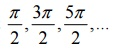1.2 Problems Based On Laplace Transform  Sufficient Conditions For Existence

1. Show that t2 is of exponential order.

Solution:

Given f(t) = t2

By the definition of exponential order,Hence t2 is of exponential order.

2. Show that the function the following function is not of exponential order  f (t ) =et2

Solution:

Given f(t) = et 2

By the definition of exponential order,1.3 Define function of class A

A function which is sectionally continuous over any finite interval and is of exponential order is known as a function of class A

2 Transforms Of Elementary Functions- Basic Properties

Important Results2.1 Problems Based On Transforms Of Elementary Functions- Basic Properties

1. Find  L[t5 + e3t + 5e-2t]

Solution:2.       Find L[sin 2t+ cos πt – 8cosh 7t +sinh bt]

Solution:2.2 First Shifting Theorem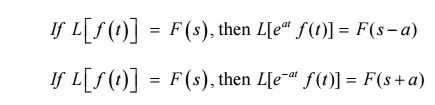2.3 Second Shifting Theorem2.4 Problems Based On First And Second Shifting Theorem

1. Find L[tn e-at]

Solution: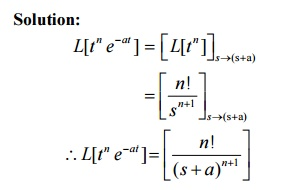2.   Find L[eat sinh bt]

Solution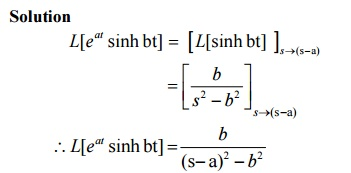2.5 Tutorial Problems:

1.    Find L[cos 4t sin 2t]

2.    Find L[sinh22t]

3.    Find L[cos(3t-4)

4.    Find L[e-tt9]

3 Transforms Of Derivatives And Integrals Of Functions

Properties:

L[ f '( t )] =s L[ f ( t )]  -(0)

L[ f ''()] =s2 L[ f ()]  -s f (0)   -f'(0)

3.1 Transform of integrals3.2 Derivatives of transform3.3 Problems Based On Derivatives Of Transform

1.  Find L[t sin at]

Solution:

We know that3.4  Tutorial Problems:

1.    Find L[t sin2t]

2.    Find L[t cos at]

3.    Find L[t sin3t cos2t]

3.5 Problems Based On Integrals Of Transform3.6 Tutorial Problems:4 Transforms Of Unit Step Function And Impulse Function

4.1 Problems Based On Unit Step Function (Or) Heaviside’s Unit Step Function

1. Define the unit step function.

Solution:

The unit step function, also called Heaviside’s unit function is defined asThis is the unit step functions at t = a. It can be also denoted by H(t-a).

2. Give the L.T of the unit step function.

Solution:

The L.T. of the unit step function is given by4.2 Tutorial Problems:

1.    Give the L.T. of the Dirac Delta function

2.    Find the L.T. of t U(t-9).

3.    Find L-1

5 Transform Of Periodic Functions

Definition: (Periodic)

A function f(x) is said to be “periodic” if and only if f(x+p) = f(x) is true for some value of p and every value of x. The smallest positive value of p for which this equation is true for every value of x will be called the period of the function.

The Laplace Transformation of a periodic function f(t) with period p given by5.1 Problems Based On Transform Of Periodic Functions

1.       Find the Laplace Transform of the Half-sine wave rectifier function5.2 Tutorial Problems:

1.   Find the Laplace Transform of triangular wave function2.       Find the Laplace transform of the square wave function (Meoander function) of period ‘a’ defined as6 Inverse Laplace Transform

a.If L[f(t)] = F(s), then L1[F(s)] = f(t) where L1 is called the inverse Laplace transform operator.

b.If F1(s) and F2(s) are L.T. of f(t) and g(t) respectively thenImportant Formulas6.1 Problems based on Inverse Laplace Transform6.2 Inverse Laplace Transforms of derivatives of F(s)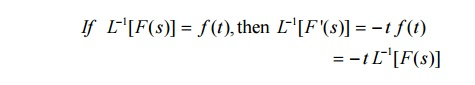6.3Problems based on Inverse Laplace Transforms of derivatives of F(s)6.4 Inverse Laplace Transform of Integrals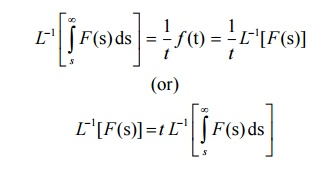6.5 Problems based on Inverse Laplace Transform of Integrals6.6 Problems based on Partial fractions method6.7 Second Shifting property6.8 Problems based on second shifting property6.9 Tutorial Problems:

a. Find the inverse L.T of Derivatives.6.10 Change of scale property6.11 Problems based on Change of scale property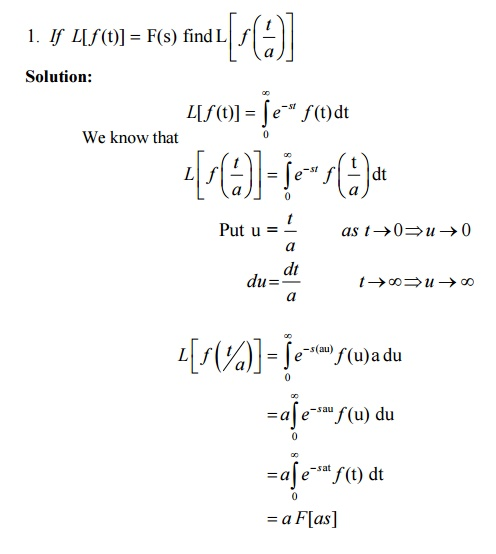6.12 Tutorial Problems:

1. If  L[ f (t)]  = F(s) then L[F(t/2) = 2 F(2s)7 Convolution Theorem

If  f (t) and g(t) are functions defined for t > = 0,

then L[ f (t ) * g (t )] = L[ f ( t )]         L[-g ( t)]

7.1 Problems on Convolution Theorem 1.Define convolution

The convolution of two functions f(t) and g(t) is defined asNote: Convolution Integral or Falting integral7.2 Tutorial Problems:

Find the inverse Laplace Transform using convolution theorem.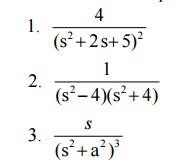8 Initial and final value theorems

8.1 Initial value theorem8.2 Final value theorem8.3 Problems based on initial value and final value theoremsL.H.S = R.H.S

Final value theorem verified.

8.4 Tutorial Problems:

Verify the initial and final value theorems for the functions9. Problems based on solution of linear ODE of second order with constant coefficients9.2 Tutorial Problems:Study Material, Lecturing Notes, Assignment, Reference, Wiki description explanation, brief detail
Mathematics (maths) : Laplace Transformation : Laplace Transformation |

Related Topics